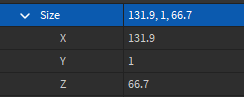# Fit vector3 inside boundary/area

how would u fit a vector3 inside a area
https://gyazo.com/3a6da13a686f4c1e40f4fd668665b9a1 like stop the part from going out of bounds of the rectangle

``````local Player = game:GetService("Players").LocalPlayer
local Character = Player.Character or Player.CharacterAdded:Wait()
local Humanoid = Character:WaitForChild("Humanoid")

local Part = Instance.new("Part", workspace)
Part.Anchored = true
Part.CanCollide = false

game:GetService("RunService").RenderStepped:Connect(function()
if Humanoid then
Part.Position = Character.PrimaryPart.Position + ((Humanoid.MoveDirection.Unit) * 5)
end
end)
``````

edit: pretty much the furthest the part could go is at the edge of the rectangle / stopping at the edge

2 Likes

How big is the rectangle part?1 Like

Try this code out:

``````local PS = game:GetService("Players")
local RNS = game:GetService("RunService")

local player = PS.LocalPlayer
local char = player.Character or player.CharacterAdded:Wait()
local hum = char:WaitForChild("Humanoid")
local boundary = -- insert boundary part

local part = Instance.new("Part", workspace) do
part.Anchored = true
part.CanCollide = false
end

local function IsPartInBoundary(pos1, size1, pos2, size2)
local p1, p2 = pos1, pos2
local s1, s2 = size1/2, size2/2

local minX1, minX2 = p1.X - s1.X, p2.X - s2.X
local maxX1, maxX2 = p1.X + s1.X, p2.X + s2.X
local minY1, minY2 = p1.Y - s1.Y, p2.Y - s2.Y
local maxY1, maxY2 = p1.Y + s1.Y, p2.Y + s2.Y
local minZ1, minZ2 = p1.Z - s1.Z, p2.Z - s2.Z
local maxZ1, maxZ2 = p1.Z + s1.Z, p2.Z + s2.Z

return
(minX1 >= minX2 and maxX1 <= maxX2) and
(minY1 >= minY2 and maxY1 <= maxY2) and
(minZ1 >= minZ2 and maxZ1 <= maxZ2)
end

RNS.RenderStepped:Connect(function()
if hum then
local pos = char.PrimaryPart.Position + ((hum.MoveDirection.Unit) * 5)

if IsPartInBoundary(pos, part.Size, boundary.Position, boundary.Size) then
part.Position = pos
end
end
end)
``````

ok i didnt specify but if the part isnt in the boundary i want it stay like on the edge and move along the player

1 Like

What do you mean by that? Does my code not already do that?

no in your code the part wont move if the part is out of the boundary, I want it to have the part follow player but not exit the boundary so it looks like it stays on the edge if its out of the boundary

Use `math.min` or `math.max` to clamp a value to a minimum/maximum value,

``````local X = math.min(Part.Position.X, 10) --Returns part's X axis position or 10 (whichever is the smallest).
``````

how would i use this here

Oh so like, you want it to move aswell like normal but also adjusts the position so that it isn’t outside of the boundary?

Yea thats what I want

1 Like

Here, try this:

``````local PS = game:GetService("Players")
local RNS = game:GetService("RunService")

local player = PS.LocalPlayer
local char = player.Character or player.CharacterAdded:Wait()
local hum = char:WaitForChild("Humanoid")
local boundary = workspace.Part

local part = Instance.new("Part", workspace) do
part.Anchored = true
part.CanCollide = false
end

local function IsPartInBoundary(pos1, size1, pos2, size2)
local p1, p2 = pos1, pos2
local s1, s2 = size1/2, size2/2

local minX1, minX2 = p1.X - s1.X, p2.X - s2.X
local maxX1, maxX2 = p1.X + s1.X, p2.X + s2.X
local minY1, minY2 = p1.Y - s1.Y, p2.Y - s2.Y
local maxY1, maxY2 = p1.Y + s1.Y, p2.Y + s2.Y
local minZ1, minZ2 = p1.Z - s1.Z, p2.Z - s2.Z
local maxZ1, maxZ2 = p1.Z + s1.Z, p2.Z + s2.Z
local afX, afY, afZ = (minX1 >= minX2 and maxX1 <= maxX2), (minY1 >= minY2 and maxY1 <= maxY2), (minZ1 >= minZ2 and maxZ1 <= maxZ2)

return afX, afY, afZ

end

RNS.RenderStepped:Connect(function()
if hum and hum.MoveDirection ~= Vector3.zero then
local pos = char.PrimaryPart.Position + ((hum.MoveDirection.Unit) * 5)
local x,y,z = IsPartInBoundary(pos, part.Size, boundary.Position, boundary.Size)

part.Position = Vector3.new(if x then pos.X else part.Position.X, if y then pos.Y else part.Position.Y, if z then pos.Z else part.Position.Z)
end
end)
``````

its perfect although there is a bug if the player spawns outside of the boundary

edit: yea its really buggy if the player spawns outside the boundary how would i fix that?

1 Like

Huh, it’s pretty weird. I’m not really sure why it’s doing that. A workaround you can do is to spawn the part inside the boundary. For some reason, if the game starts with the part inside the boundary, it doesn’t glitch out.